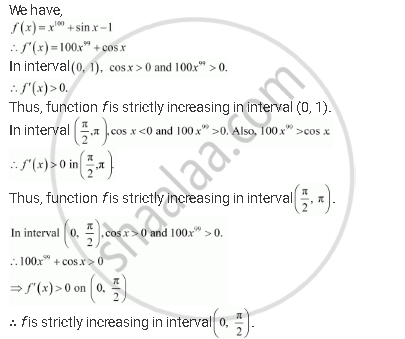Share

# On Which of the Following Intervals is the Function F Given Byf(X) =X100 + Sin X –1 Strictly Decreasing? - CBSE (Science) Class 12 - Mathematics

ConceptIncreasing and Decreasing Functions

#### Question

On which of the following intervals is the function f given byf(x) =x100 + sin x –1 strictly decreasing?

(A) (0,1)

(B) (pi/2, pi)

(C) (0, pi/2)

(D) None of these

#### SolutionHence, function f is strictly decreasing in none of the intervals.